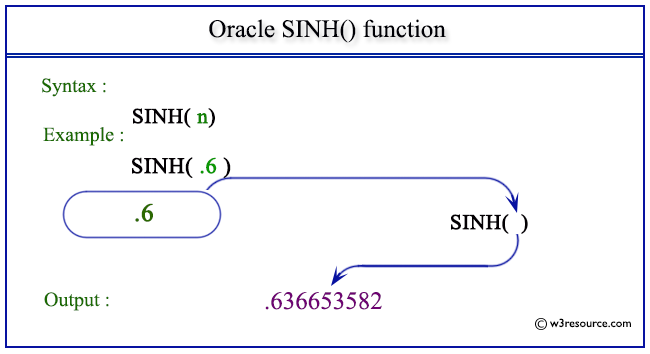# Oracle SINH() function

## Description

This SINH() function returns the hyperbolic sine of n (an angle expressed in radians).
The function takes any numeric or nonnumeric data type (can be implicitly converted to a numeric data type) as an argument.
If the argument is BINARY_FLOAT, then the function returns BINARY_DOUBLE. Otherwise, the function returns the same numeric data type as the argument.

Syntax:

`SIN(n)`

Parameters:

Name Description
n A number whose sine value is to be retrieved.

Pictorial Presentation of SINH() functionExample:

``````SELECT SINH(.6)  "Hyperbolic sine of .6" FROM dual;
```
```

Here is the result.

```Hyperbolic sine of .6
---------------------
.636653582
```

The above statement will return the sine of .6.

Previous: SIN
Next: SQRT

﻿